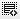## Calculation of ROI0
Hello Binny,
I have not completely understood the two quiz questions based on ROI calculation. I have also gone through the solution that you have given to other people. According to the example given in the video, I have calculated the ROI in each case but i want to know why does it differ from the answers? Do we need to remove any factor after calculating the ROI?0

Akanksha the ROI formula should be (Gain-Cost)/Cost.
So if you invested \$50 and got back \$150 then ROI will be (150-50)/50 = 200%. From the Gain you need to subtract any debt and interest payback if any.

Click on thisicon to add code snippet.